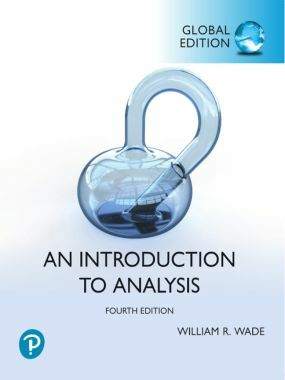# An Introduction to Analysis William Wade 4th GE 9781292357874

RM 105.00

TITLE : An Introduction to Analysis William Wade

ISBN13 : 9781292357874

PUBLISHER : PEARSON (2021)

EDITION : 4TH  GLOBAL EDITION PAPERBACK

PAGES : 696 PAGES

For one- or two-semester junior or senior level courses in Advanced Calculus, Analysis I, or Real Analysis.

This title is part of the Pearson Modern Classics series.

This text prepares students for future courses that use analytic ideas, such as real and complex analysis, partial and ordinary differential equations, numerical analysis, fluid mechanics, and differential geometry. This book is designed to challenge advanced students while encouraging and helping weaker students. Offering readability, practicality and flexibility, Wade presents fundamental theorems and ideas from a practical viewpoint, showing students the motivation behind the mathematics and enabling them to construct their own proofs.

Part I. ONE-DIMENSIONALTHEORY

1. The Real Number System

1.1 Introduction

1.2 Ordered field axioms

1.3 Completeness Axiom

1.4 Mathematical Induction

1.5 Inverse functions and images

1.6 Countable and uncountable sets

2. Sequences in R

2.1 Limits of sequences

2.2 Limit theorems

2.3 Bolzano-Weierstrass Theorem

2.4 Cauchy sequences

*2.5 Limits supremum and infimum

3. Functions on R

3.1 Two-sided limits

3.2 One-sided limits and limits atinfinity

3.3 Continuity

3.4 Uniform continuity

4. Differentiability on R

4.1 The derivative

4.2 Differentiability theorems

4.3 The Mean Value Theorem

4.4 Taylor's Theorem and l'Hopital'sRule

4.5 Inverse function theorems

5 Integrability on R

5.1 The Riemann integral

5.2 Riemann sums

5.3 The Fundamental Theorem ofCalculus

5.4 Improper Riemann integration

*5.5 Functions of boundedvariation

*5.6 Convex functions

6. Infinite Series of Real Numbers

6.1 Introduction

6.2 Series with nonnegative terms

6.3 Absolute convergence

6.4 Alternating series

*6.5 Estimation of series

7. Infinite Series of Functions

7.1 Uniform convergence ofsequences

7.2 Uniform convergence of series

7.3 Power series

7.4 Analytic functions

*7.5 Applications

Part II. MULTIDIMENSIONAL THEORY

8. Euclidean Spaces

8.1 Algebraic structure

8.2 Planes and lineartransformations

8.3 Topology of Rn

8.4 Interior, closure, and boundary

9. Convergence in Rn

9.1 Limits of sequences

9.2 Heine-Borel Theorem

9.3 Limits of functions

9.4 Continuous functions

*9.5 Compact sets

*9.6 Applications

10. Metric Spaces

10.1 Introduction

10.2 Limits of functions

10.3 Interior, closure, boundary

10.4 Compact sets

10.5 Connected sets

10.6 Continuous functions

10.7 Stone-Weierstrass Theorem

11. Differentiability on Rn

11.1 Partial derivatives andpartial integrals

11.2 The definition ofdifferentiability

11.3 Derivatives, differentials, andtangent planes

11.4 The Chain Rule

11.5 The Mean Value Theorem andTaylor's Formula

11.6 The Inverse Function Theorem

*11.7 Optimization

12. Integration on Rn

12.1 Jordan regions

12.2 Riemann integration on Jordanregions

12.3 Iterated integrals

12.4 Change of variables

*12.5 Partitions of unity

*12.6 The gamma function andvolume

13. Fundamental Theorems of VectorCalculus

13.1 Curves

13.2 Oriented curves

13.3 Surfaces

13.4 Oriented surfaces

13.5 Theorems of Green and Gauss

13.6 Stokes's Theorem

*14. Fourier Series

*14.1 Introduction

*14.2 Summability of Fourierseries

*14.3 Growth of Fouriercoefficients

*14.4 Convergence of Fourierseries

*14.5 Uniqueness

Appendices

A. Algebraic laws

B. Trigonometry

C. Matrices and determinants